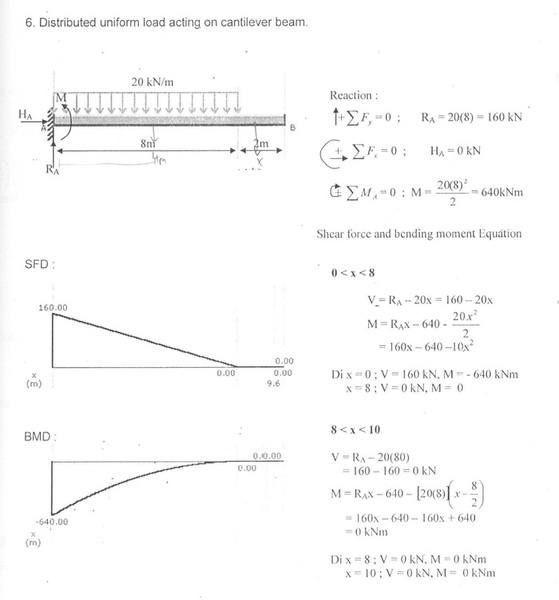# Shear force and moment

## Homework Statement

in the book , i was told that when the shear force is 0 , moment is maximum . However , in this question , i found that when the shear foce is 0 , the moment is also 0 . I'm wondering is the ststemnet true ?SteamKing
Staff Emeritus
Homework Helper

## Homework Statement

in the book , i was told that when the shear force is 0 , moment is maximum . However , in this question , i found that when the shear foce is 0 , the moment is also 0 . I'm wondering is the ststemnet true ?## The Attempt at a Solution

Obviously, if the beam is a cantilever, the shear force and the bending moment can both be zero at the free end. You won't find the maximum moment there in any event.

I think what the author was trying to say was that since the derivative of the bending moment, dM/dx = V, the locations where the shear is zero are also possible locations where the bending moment has a relative maximum or minimum.

Obviously, if the beam is a cantilever, the shear force and the bending moment can both be zero at the free end. You won't find the maximum moment there in any event.

I think what the author was trying to say was that since the derivative of the bending moment, dM/dx = V, the locations where the shear is zero are also possible locations where the bending moment has a relative maximum or minimum.
so , for the above case , shear force = 0 , moment = 0(minimum) ?

SteamKing
Staff Emeritus
•